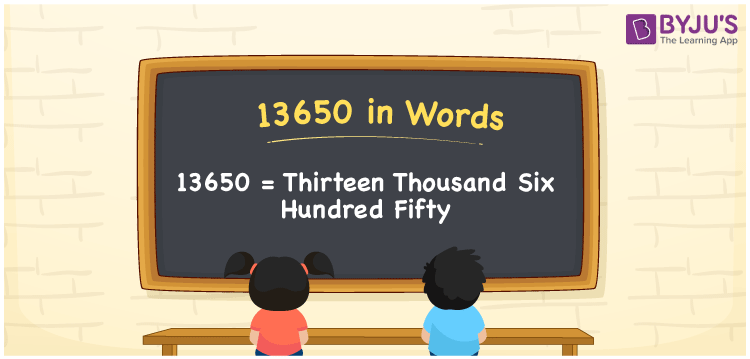# 13650 in Words

Thirteen Thousand Six Hundred Fifty is the word form of the number 13650. It will be easy to convert any number into words using a place value of a number. Hence, we need a place value chart to create numerical names. If you bought an oven for Rs. 13650, you can say, “I bought an oven worth Rupees Thirteen Thousand Six Hundred Fifty”. The number 13650 is a cardinal number. Let us learn how to read and write 13650 in English words in this article.

 13650 in Words Thirteen Thousand Six Hundred Fifty Thirteen Thousand Six Hundred Fifty in Numerical Form 13650

## 13650 in English Words

English alphabet is essential to express numbers in words. Hence, we can write 13650 in words as Thirteen Thousand Six Hundred Fifty.## How to Write 13650 in Words?

To write the number name, first, we have to determine the position of each digit in a given number. The following chart shows the place value chart for the number 13650.

 Ten Thousands Thousands Hundreds Tens Ones 1 3 6 5 0

Hence, we can write the expanded form as:

1 x Ten Thousand + 3 x Thousand + 6 x Hundred + 5 x Ten + 0 x One

= 1 x 10000 + 3 x 1000 + 6 x 100 + 5 x 10 + 0 x 1

= 10000 + 3000 + 600 + 50 + 0

= 10000 + 3000 + 600 + 50

= 13650

= Thirteen Thousand Six Hundred Fifty

Thus, 13650 in words is written as Thirteen Thousand Six Hundred Fifty.

Interesting way of writing 13650 in words

1 = One

13 = Thirteen

136 = One Hundred and Thirty-Six

1365 = One Thousand Three Hundred Sixty-Five

13650 = Thirteen Thousand Six Hundred Fifty

Hence, the word form of the number 13650 is Thirteen Thousand Six Hundred Fifty

13650 is a natural number that is the successor of 13649 and the predecessor of 13651

• 13650 in words – Thirteen Thousand Six Hundred Fifty
• Is 13650 an odd number? – No
• Is 13650 an even number? – Yes
• Is 13650 a perfect square number? – No
• Is 13650 a perfect cube number? – No
• Is 13650 a prime number? – No
• Is 13650 a composite number? – Yes

## Frequently Asked Questions on 13650 in Words

Q1

### How to write 13650 in words?

13650 in words is written as Thirteen Thousand Six Hundred Fifty.
Q2

### Simplify 12000 + 1650, and express in words.

Simplifying 12000 + 1650, we get 13650. Hence, 13650 in words is Thirteen Thousand Six Hundred Fifty.
Q3

### 13650 is a composite number. True or False.

True, 13650 is a composite number.
Test your Knowledge on 13650 in Words